Electron. J. Diff. Eqns., Vol. 2008(2008), No. 61, pp. 1-12.

### Three solutions for singular p-Laplacian type equations Zhou Yang, Di Geng, Huiwen Yan

Abstract:
In this paper, we consider the singular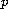-Laplacian type equation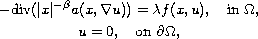where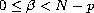,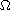is a smooth bounded domain in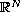containing the origin,satisfies some growth and singularity conditions. Under some mild assumptions on, applying the three critical points theorem developed by Bonanno, we establish the existence of at least three distinct weak solutions to the above problem ifadmits some hypotheses on the behavior at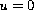or perturbation property.

Submitted January 14, 2008. Published April 22, 2008.
Math Subject Classifications: 35J60.
Key Words: p-Laplacian operator; singularity; multiple solutions.

Show me the PDF file (264 KB), TEX file, and other files for this article.Zhou Yang School of Math. Sci., South China Normal University Guangzhou 510631, China email: yangzhou@scnu.edu.cn Di Geng School of Math. Sci., South China Normal University Guangzhou 510631, China email: gengdi@scnu.edu.cn Huiwen Yan School of Math. Sci., South China Normal University Guangzhou 510631, China email: hwyan10@yahoo.com.cn